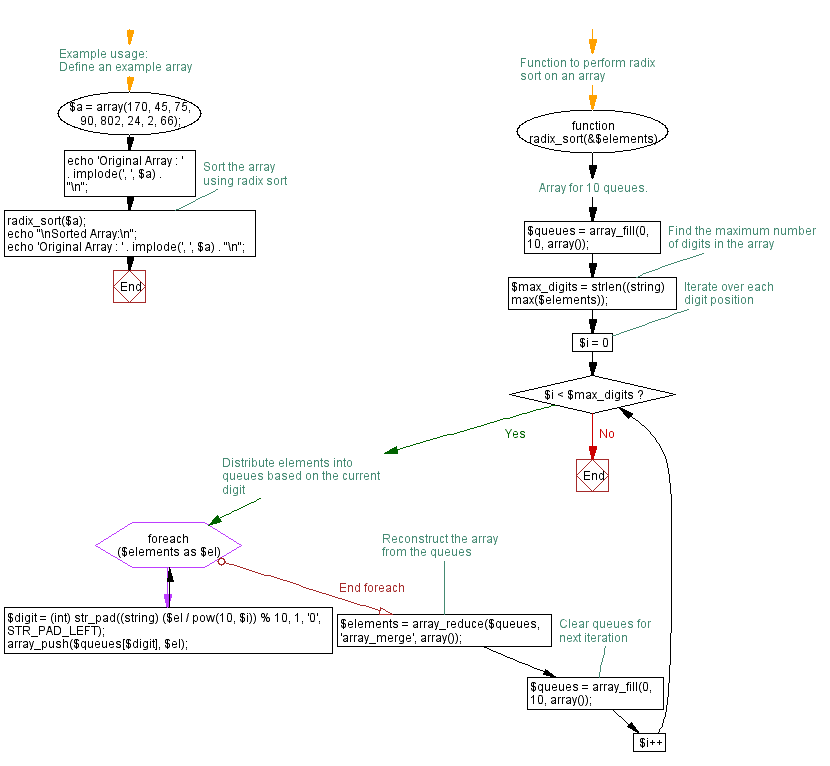# JavaScript Sorting Algorithm: Flash sort

## JavaScript Sorting Algorithm: Exercise-12 with Solution

Write a JavaScript program to sort a list of elements using Flash sort.

Flashsort is a distribution sorting algorithm showing linear computational complexity O(n) for uniformly distributed data sets and relatively little additional memory requirement. The original work was published in 1998 by Karl-Dietrich Neubert.
The basic idea behind flashsort is that in a data set with a known distribution, it is easy to immediately estimate where an element should be placed after sorting when the range of the set is known.

Sample Solution: -

HTML Code:

``````<!DOCTYPE html>
<html>
<meta charset="utf-8">
<title>JavaScript program of Flash sort</title>
<body></body>
</html>
```
```

JavaScript Code:

``````function flash_sort(arr)
{
var max = 0, min = arr;
var n = arr.length;
var m = ~~(0.45 * n);
var l = new Array(m);

for (var i = 1; i < n; ++i) {
if (arr[i] < min) {
min = arr[i];
}
if (arr[i] > arr[max]) {
max = i;
}
}

if (min === arr[max]) {
return arr;
}

var c1 = (m - 1) / (arr[max] - min);

for (var k = 0; k < m; k++) {
l[k] = 0;
}
for (var j = 0; j < n; ++j) {
k = ~~(c1 * (arr[j] - min));
++l[k];
}

for (var p = 1; p < m; ++p) {
l[p] = l[p] + l[p - 1];
}

var hold = arr[max];
arr[max] = arr;
arr = hold;

//permutation
var move = 0, t, flash;
j = 0;
k = m - 1;

while (move < (n - 1)) {
while (j > (l[k] - 1)) {
++j;
k = ~~(c1 * (arr[j] - min));
}
if (k < 0) break;
flash = arr[j];
while (j !== l[k]) {
k = ~~(c1 * (flash - min));
hold = arr[t = --l[k]];
arr[t] = flash;
flash = hold;
++move;
}
}

//insertion
for (j = 1; j < n; j++) {
hold = arr[j];
i = j - 1;
while (i >= 0 && arr[i] > hold) {
arr[i + 1] = arr[i--];
}
arr[i + 1] = hold;
}
return arr;
}
var arra = [3, 0, 2, 5, -1, 4, 1];
console.log("Original Array Elements");
console.log(arra);
console.log("Sorted Array Elements");
console.log(flash_sort(arra, 0, 5));
```
```

Sample Output:

```Original Array Elements
[3,0,2,5,-1,4,1]
Sorted Array Elements
[-1,0,1,2,3,4,5]
```

Flowchart:Live Demo:

See the Pen searching-and-sorting-algorithm-exercise-12 by w3resource (@w3resource) on CodePen.

Improve this sample solution and post your code through Disqus

What is the difficulty level of this exercise?

Test your Programming skills with w3resource's quiz.

﻿